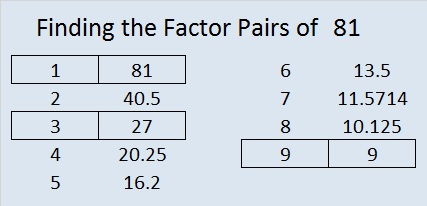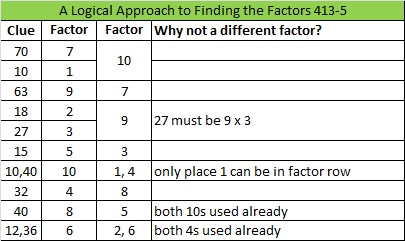# 81 and Level 5This week’s puzzles and last week’s solutions: 10 Factors 2014-03-31

81 is a composite number and is 9 squared. 81 = 1 x 81, 3 x 27, or 9 x 9. Factors of 81: 1, 3, 9, 27, 81. Prime factorization: 81 = 3 x 3 x 3 x 3 which can also be written 3⁴.Since √81 = 9, a whole number, 81 is a perfect square.

Sometimes 81 is a clue in the FIND THE FACTORS puzzles. Even though it has other factors, the only multiplication fact we use is 9 x 9 = 81.